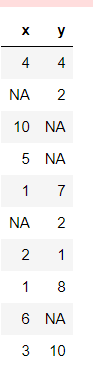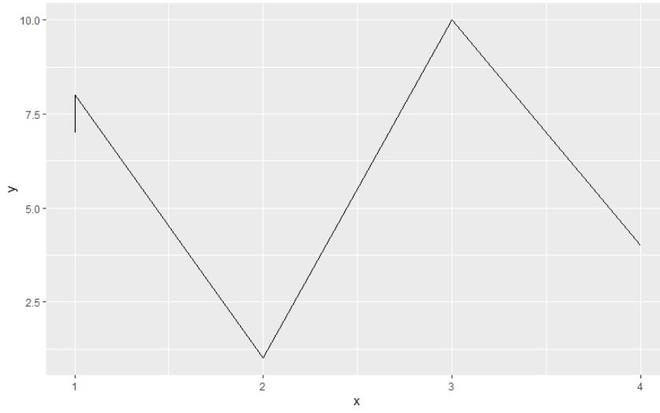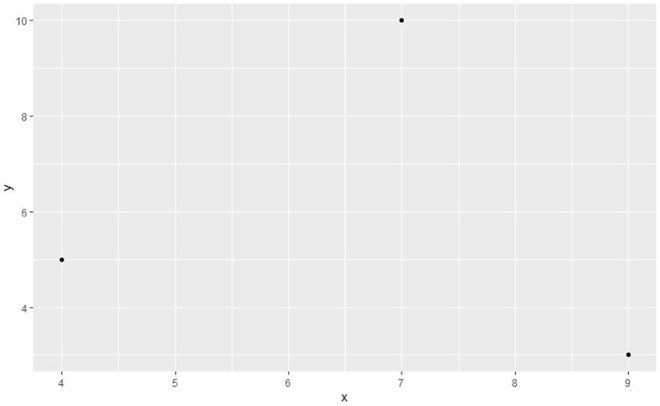Remove NA Values from ggplot2 Plot in R

• Last Updated : 23 Aug, 2021

In this article, we are going to see how to remove the NA values from the ggplot2 plot in the R programming language.

Using complete.cases() function

complete.cases() function: This function will be returning a logical vector indicating which cases are complete, i.e., have no missing values.

Syntax: complete.cases(…)

Arguments:

• …: a sequence of vectors, matrices, and data frames.

Create dataframe with NA values for demonstration:

Python3

 library("ggplot2")data <- data.frame(x = c(4,NA, 10,5, 1, NA, 2, 1, 6, 3),                   y = c(4, 2, NA, NA, 7, 2, 1, 8,NA,10))data

Output:Example 1: Removing NA values from plot

In this example, we will be plotting a ggplot2 line plot of 10 data points and further with the help of the complete.cases() function we will be removing the NA value to plot the ggplot2 line plot in the R programming language.

R

 library("ggplot2")  data <- data.frame(x = c(4,NA, 10,5, 1, NA, 2, 1, 6, 3),                   y = c(4, 2, NA, NA, 7, 2, 1, 8,NA,10))  data_complete=data[complete.cases(data), ]ggplot(data_complete, aes(x, y)) +geom_line()

Output:Example 2: Removing NA values from plot with a different dataset

In this example, we will be plotting a ggplot2 plot of 5data points and further with the help of the complete.cases() function we will be removing the NA value to plot the ggplot2 plot in the R programming language.

R

 library("ggplot2")  data <- data.frame(x =c(7,9,NA,4,NA),                   y=c(10,3,8,5,6) )  data_complete = data[complete.cases(data), ]ggplot(data_complete, aes(x, y)) +geom_point()

Output:My Personal Notes arrow_drop_up